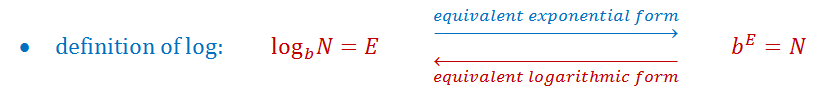# Solving exponential equations with logarithms

### Solving exponential equations with logarithms

#### Lessons• 1.
Solve for $x$ , by converting from exponential form
to log form:
a)
$y = 5 \cdot {2^x}$

b)
$6 \cdot {3^x} = 90$

• 2.
Let's revisit the earlier example about Bianca saving up money to buy her dream car, Mini Cooper.
Now we are ready to find out the exact amount of time it'll take Bianca to save up $30,000: On Bianca's 5-year-old birthday, Bianca gets a birthday gift of$100 cash from her dad.
Bianca's dad promises to double her money yearly if Bianca plans to not spend the